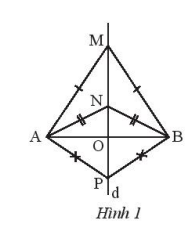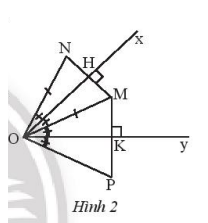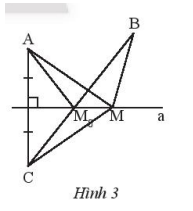## Solving SBT Lesson 5: The perpendicular bisector of a line segment (C8 SBT Math 7 Horizon) – Math Book

SBT Solution Lesson 5: The perpendicular bisector of a line segment (C8 SBT Math 7 Horizon)
===========

### Solution 1 page 55 SBT Math 7 Creative horizon episode 2 – CTST

Given three isosceles triangles MAB, NAB, PAB with common base AB. Prove that the three points M, N, and P are collinear.

Detailed instructions for solving Lesson 1

Solution method

Prove that the three points M, N, and P all belong to the median line of AB.

Detailed explanationWe have isosceles triangles MAB, NAB, PAB with the same base AB, infer MA = MB, NA = NB,

PA = PB. Points M, N, and P belong to the perpendicular bisector of AB, so they are collinear.

–>

— *****

### Solution 2 page 55 SBT Math 7 Creative horizon episode 2 – CTST

Let angle xOy equal $${45^o}$$ and point M in the angle xOy. Draw a point N such that Ox is the perpendicular bisector of MN, draw a point P such that Oy is the perpendicular bisector of MP.

a) Prove ON = OP.

b) Calculate the measure of angle NOP

Detailed instructions for solving Lesson 2

Solution method

Use the property of the median line to prove ON = OP

Detailed explanationa) We have Ox as the perpendicular bisector of MN, so OM = ON

We have Oy as the median of MP, so OM = OP.

So ON = OP.

b) Let H and K be the midpoints of MN and MP, respectively, so HM = HN ; KM = KP

We have: $$\Delta OHM = \Delta OHN$$ (because OH is common, OM = ON, HM = HN)

$$\Delta OKM = \Delta OKP$$ (because of general OK, OM = OP, KM = KP)

Derive: $$\widehat {NOP} = \widehat {NOM} + \widehat {MOP} = 2\left( {\widehat {xOM} + \widehat {MOy}} \right) = 2\widehat {xOy} = {2.45^o} = {90^o}$$

–>

— *****

### Solve problem 3 page 55 SBT Math 7 Creative horizon episode 2 – CTST

Given two points A, B are the positions of the two factories on the same side of the river as the straight line a. Draw point C such that a is the perpendicular bisector of AC. Take an arbitrary point M on a.

a) Prove MA + MB $$\ge$$ BC

b) Find the location of the location $${M_o}$$ on the river bank to build a pumping station so that the total length of the pipeline from the pumping station to the two plants is the shortest.

Detailed instructions for solving Lesson 3

Solution method

Using with arbitrary points M, B, C we always have: MB + MC $$\ge$$ BC to deduce the proof in the problem and find the position $${M_o}$$

Detailed explanationa) We have a point M on the perpendicular bisector of AC, so MA = MC.

With three arbitrary points M, B, C we always have:

$$MB + MC \ge BC$$

So $$MA + MB \ge BC$$

b) We have: $$MA + MB \ge BC$$, deduce the shortest MA + MB when B < C, M is collinear.

So the point $${M_o}$$, to find is the intersection of line BC and line a.

–>

— *****